# what are the characteristics of arithmetic mean ?

MEANING OF ARITHMATIC MEAN :

The arithmetic mean of a data set is defined to be the sum of all the observations of the data set divided by the total number of observations in the data set.

If  be the values assumed by a variable  then their arithmetic mean or A.M. is defined as:

PROPERTIES OF ARITHMATIC MEAN :

1. The sum of the deviations, of all the values of x, from their arithmetic mean, is zero.

Justification :Since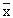is a constant,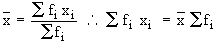2. The product of the arithmetic mean and the number of items gives the total of all items.

Justification :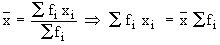or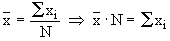3. If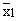andare the arithmetic mean of two samples of sizes n1 and n2respectively then, the arithmetic meanof the distribution combining the two can be calculated as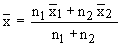This formula can be extended for still more groups or samples.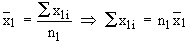Justification := total of the observations of the first sample

Similarly= total of the observations of the first sample

The combined mean of the two samples

=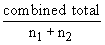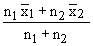• 0
What are you looking for?